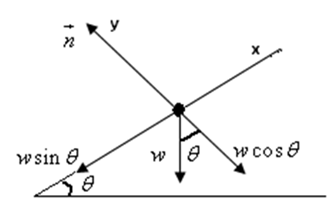Problem

a. A 0.60 kg bullfrog is sitting at rest on a level log. How large is the normal force o...

a. A 0.60 kg bullfrog is sitting at rest on a level log. How large is the normal force of the log on the frog?

b. A second 0.60 kg bullfrog is on a log tilted 30° above horizontal. How large is the normal force of the log on this frog?

Step-by-Step Solution

Solution 1

An object is said to be in stable equilibrium when the net force on the object is equal to zero. The term net force indicates that the vector sum of all the forces acting simultaneously on the object is equal to zero.

(a)

In the given scenario, the bullfrog is resting on a level log. In this case, the weight $$w$$ of the bullfrog acts vertically downwards and the normal force $$n$$ acts vertically upwards.

As the bullfrog is on the log is at rest, the net force $$F_{\text {nd }}$$ on it must be zero.

From Newton's second law,

$$F_{\text {ect }}=n-w$$

The weight $$w$$ of the bullfrog is,

$$F_{G}=m g$$

Here, $$m$$ is the mass and $$g$$ is the acceleration due to gravity.

Replace $$w$$ with $$m g$$ and $$F_{\text {oct }}$$ with 0 in $$F_{\text {et }}=n-w$$ and solve for $$n$$.

\begin{aligned} &0=n-m g \\ &n=m g \end{aligned}

Substitute $$0.60 \mathrm{~kg}$$ for $$m$$ and $$9.80 \mathrm{~m} / \mathrm{s}^{2}$$ for $$g$$.

\begin{aligned} n &=(0.60 \mathrm{~kg})\left(9.80 \mathrm{~m} / \mathrm{s}^{2}\right) \\ &=5.88 \mathrm{~N} \end{aligned}

(b)

The free body diagram of the given bullfrog is shown in the below figure.In the above figure $$\theta$$ is the angle of inclination, $$w \cos \theta$$ and $$w \sin \theta$$ are the components of the weight.

As there is motion along the vertical to the inclined plane, the net force in vertical direction is equal to zero.

From Newton's second law,

$$F_{\mathrm{nt}}=n-w \cos \theta$$

Replace wwith $$m g$$ and $$F_{\text {na }}$$ with 0 in the above equation and solve for $$n_{+}$$

\begin{aligned} &0=n-m g \cos \theta \\ &n=m g \cos \theta \end{aligned}

Substitute $$30^{\circ}$$ for $$\theta, 0.60 \mathrm{~kg}$$ for $$m$$ and $$9.80 \mathrm{~m} / \mathrm{s}^{2}$$ for $$g$$.

\begin{aligned} n &=(0.60 \mathrm{~kg})\left(9.80 \mathrm{~m} / \mathrm{s}^{2}\right) \cos 30^{\circ} \\ &=5.1 \mathrm{~N} \end{aligned}